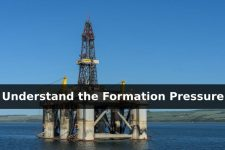Formation pressure is the pressure of fluid contained in pore space of rock and there are 3 categories of the formation pressure which are normal pressure, abnormal pressure and subnormal pressure.

1. Normal Pressure: Normal pressure is the hydrostatic of water column from the surface to the subsurface formation.  It can be simply stated that normal pressure is equal to hydrostatic pressure gradient of water in pore spaces of  formations on each area. The concentration of salt in water affects the normal pressure. Higher salt concentration in water, higher specific gravity of water will be. Therefore, the normal pressure can vary from slightly salt 0.433 psi/ft (8.33 PPG) to highly concentrated salt 0.478 psi/ft (9.2 PPG) based on salt concentration in water. Table 1 demonstrates the average normal pressure gradient based on several areas.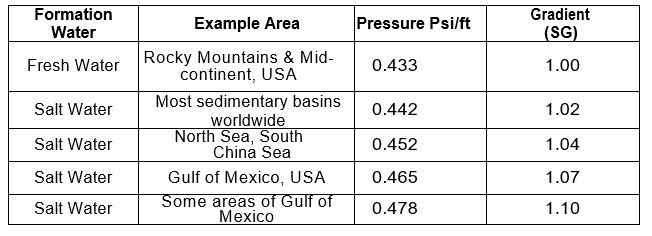Table 1 – Average Normal Pressure Gradient from Some Areas

2. Abnormal Pressure: The abnormal pressure is the pressure greater than the pressure column of water (normal pressure). Generally, the abnormal pressure zones are good reservoir which oil companies are looking for. This kind of pressure has the highest potential leading to a well control problem.

3. Subnormal Pressure: The subnormal pressure is the pressure that is less than normal pressure and it  possibly causes lost circulation problems.

Looking at the drawing below (Figure 1), it demonstrates the comparison of formation pressure when drilling into each pressure regime. At the same True Vertical Depth (TVD), subnormal pressure shows least pressure in comparison to others. However, abnormal pressure gives the highest pressure at the same level of TVD.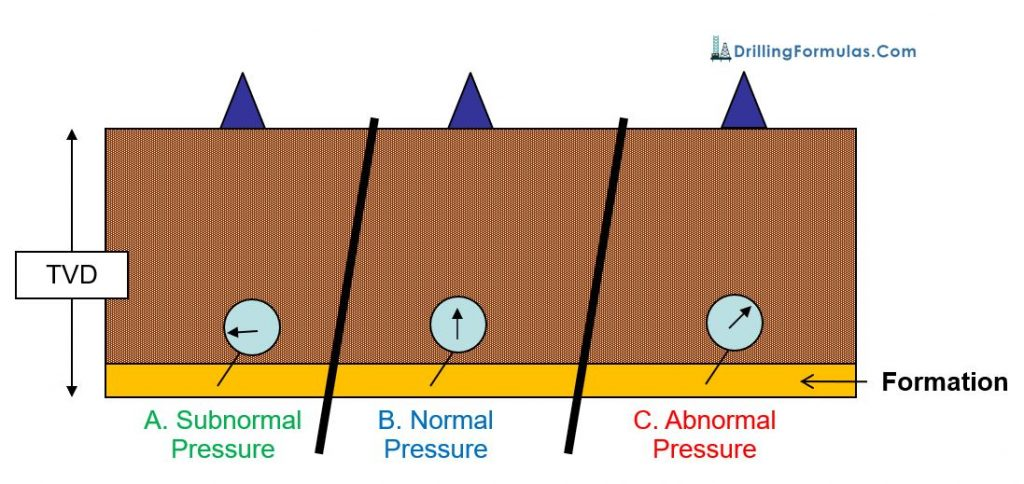Figure 1 – Simplified Formation Pressure Illustration

References

Coleman, S. (2018). Well Control Quiz Online. [online] Well Control Quiz Online – Test Your Well Control Knowledge for Free. Available at: http://wellcontrolquiz.com/ [Accessed 2 Aug. 2018].

Cormack, D. (2007). An introduction to well control calculations for drilling operations. 1st ed. Texas: Springer.

Crumpton, H. (2010). Well Control for Completions and Interventions. 1st ed. Texas: Gulf Publishing.

Grace, R. (2003). Blowout and well control handbook [recurso electrónico]. 1st ed. Paises Bajos: Gulf Professional Pub.

Grace, R. and Cudd, B. (1994). Advanced blowout & well control. 1st ed. Houston: Gulf Publishing Company.

Watson, D., Brittenham, T. and Moore, P. (2003). Advanced well control. 1st ed. Richardson, Tex.: Society of Petroleum Engineers.

## Understand Hydrostatic Pressure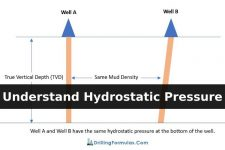In a well, any pressure created by a static column of fluid is called ‘Hydrostatic Pressure’ (HP); at any given True Vertical Depth (TVD). With ‘hydro’ representing water, which exerts pressure, ‘static’ means it has no movement. Any pressure developed by a column of fluid that isn’t moving, therefore, can be considered hydrostatic pressure; fluid in this sense can be either liquid or gas.

The relationship of hydrostatic pressure is shown in the equation below.

HP (Hydrostatic Pressure) = density x g (gravity acceleration) x h (True Vertical Depth, TVD)

In oilfield term, the formula above is modified so that people can use it easily. The formulas are as follows:

HP (Hydrostatic Pressure) = Constant x MW (Mud Weight or Mud Density)  x TVD (True Vertical Depth)

HP (psi)  = 0.052 x MW (ppg) x TVD (ft) ** Most frequent used in the oilfield **

HP (psi) = 0.007 x MW (pcf) x TVD (ft)

HP (kPa) = 0.00981 x MW (kg/m3) x TVD (m)

Depending on which unit is used for calculation, there are several conversion factors such as 0.052, 0.007, 0.00981 for instant as you can see from the equations above.

According to the equations above, Hydrostatic Pressure is not a function of hole geometry. Only mud weight and True Vertical Depth (TVD) affect on Hydrostatic Pressure. For example (a picture below); well A and well B have the same vertical depth. With the same mud density in hole, the bottom hole pressure due to hydrostatic pressure is the same. The only different between Well A and Well B is mud volume.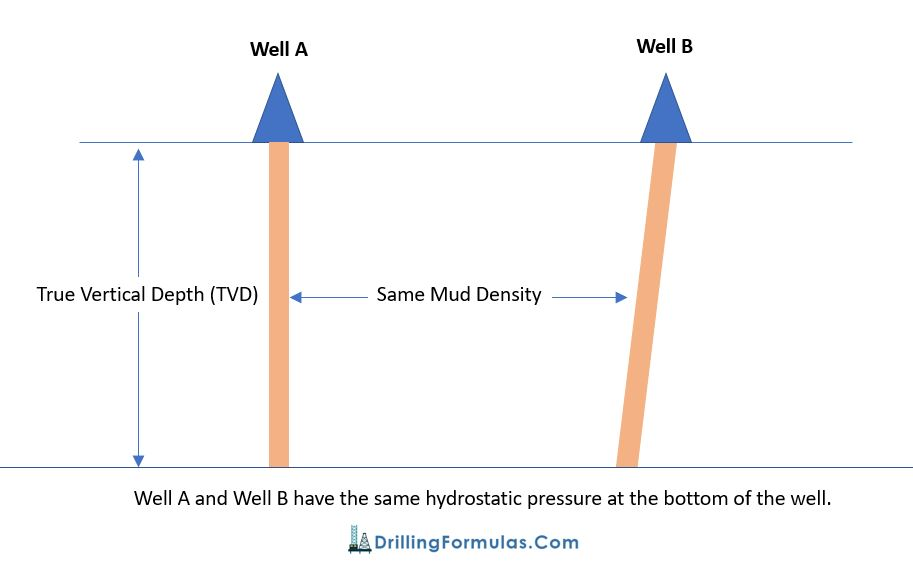This concept is basic and very important for many aspects such as well control, balance cementing, u-tube, etc.

## Pressure in a well

In a static condition

• Pressure at any depth = Hydrostatic Pressure (HP) + Surface Pressure (SP)
• Pressure between 2 points is HP between these points

The diagram below demonstrates the relationship of pressure in a well.

At point 1, Pressure@1 = Surface Pressure (SP) + Hydrostatic Pressure @ 1 (HP1)

At point 1, Pressure@2 = Surface Pressure (SP) + Hydrostatic Pressure@1 (HP1) + Hydrostatic Pressure@2 (HP2)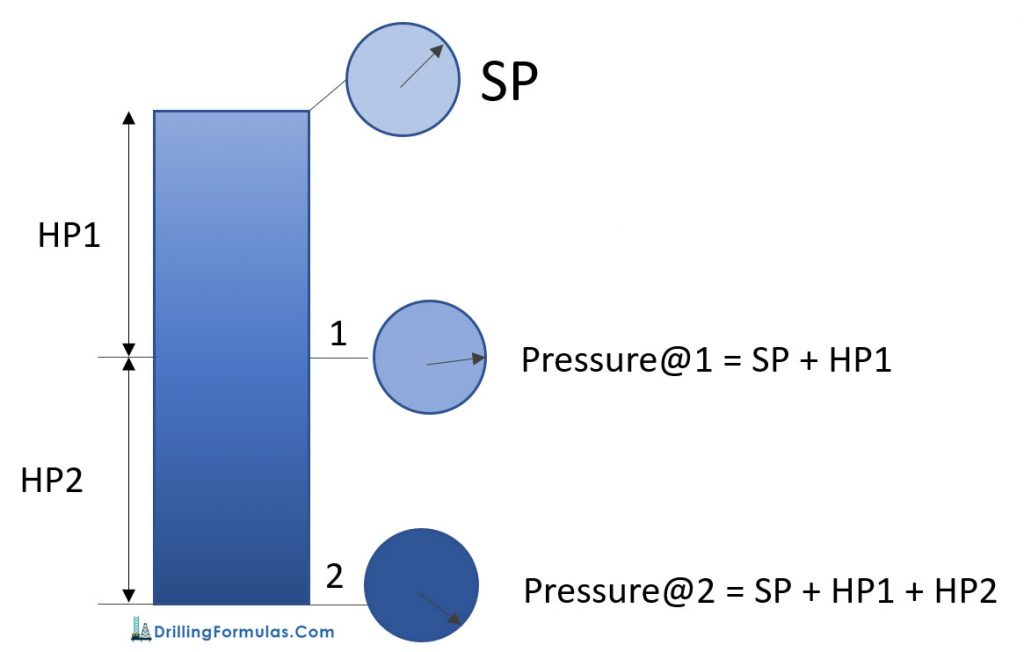Ref books:

Lapeyrouse, N.J., 2002. Formulas and calculations for drilling, production and workover, Boston: Gulf Professional publishing.

Bourgoyne, A.J.T., Chenevert , M.E. & Millheim, K.K., 1986. SPE Textbook Series, Volume 2: Applied Drilling Engineering, Society of Petroleum Engineers.

Mitchell, R.F., Miska, S. & Aadny, B.S., 2011. Fundamentals of drilling engineering, Richardson, TX: Society of Petroleum Engineers.